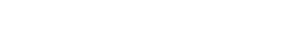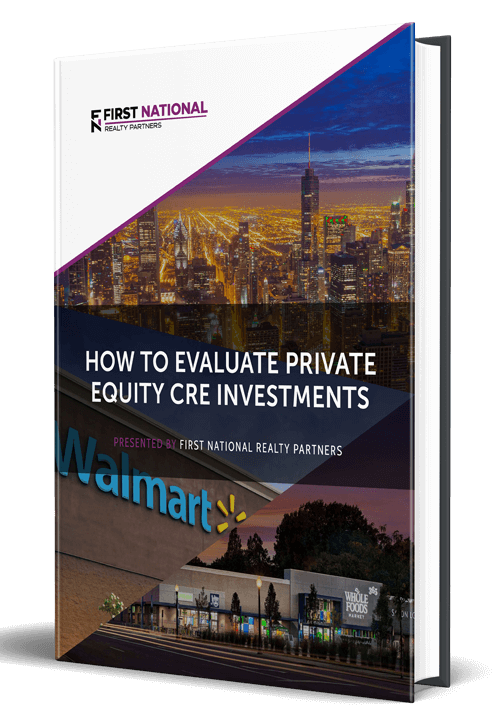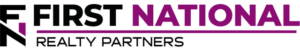# An Investor’s Guide to Levered IRR

### Key Takeaways

• Internal Rate of Return is an investment return metric that provides investors with the annual rate of return for each dollar invested, for each time period it is invested in.
• The formulas used to calculate IRR can be complex.  Instead, real estate investors should create a proforma projection of cash flows for a defined holding period and use an IRR function in a spreadsheet to calculate it.
• There are two types of IRR, unlevered and levered.  Unlevered means no debt, levered means with debt.  In most cases, the levered IRR should be higher than unlevered IRR.
• Real estate investors should know how to calculate IRR and a host of other return metrics as part of the due diligence process when evaluating a potential investment.

If an investor is going to take the risk of allocating money to a commercial real estate investment, certainly one of the major questions on their mind is how much of a return they stand to earn on their investment. There are many ways to measure this, but one of the most common, and widely accepted is known as Internal Rate of Return – IRR for short.

In this article, we are going to discuss IRR. We will describe what it is, how it is calculated, why it is important in commercial real estate, and the different varieties – one of which is known as “levered IRR.” By the end, readers will be able to calculate this important metric as part of their pre-investment due diligence process.

At First National Realty Partners, we specialize in the acquisition and management of grocery store-anchored retail centers and we always calculate potential IRR as part of our due diligence process. If you are an Accredited Investor and would like to learn more about our current investment opportunities, click here.

## What is IRR?

Internal Rate of Return is a financial metric used in both a commercial real estate (CRE) and non real estate context and there are two ways to think about it.

The first is that the Internal Rate of Return is the discount rate that sets the net present value (NPV) of a stream of cash equal to zero.

The second is that it provides investors with an idea of the expected annual rate of return on each dollar invested, for each time period it is invested in.

Or, to put it in simpler terms, IRR provides investors with an expectation of the annual return that they can earn on their investment.

## What is Levered IRR?

IRR can be used to analyze investments where leverage, or debt, is used to purchase the property.  When there is no debt involved in the transaction (an equity only deal), then we typically refer to the IRR as “unlevered”.  When leverage is involved, we call the IRR metric “levered IRR”, but it is used in the same manner as unlevered IRR to analyze the investment.

IRR (unlevered) and levered IRR are calculated the same way, as we will see below.  The only difference is that the use of debt amplifies the return that the investor sees.  So, a positive return will generally be higher when leverage is used, and a negative return will be lower.  This concept will become clear when we present an example below, and it is critical that investors understand how leverage magnifies returns – both positively and negatively.

## How Is Levered IRR Calculated?

The same formula is used to calculate IRR whether it is levered or unlevered. The calculation is complex, but investors are fortunate that they do not have to calculate it manually. Instead, they can use the “IRR” or “XIRR” functions that are built into spreadsheet programs (like Excel or Google Sheets) or financial modeling tools like Argus.

But, to solve for IRR, it is first necessary to create a financial proforma, which projects future cash flows over a planned holding period. While every deal is different, proformas typically follow the same structure and they require the input of key information like the amount of the initial investment, the cost of capital, the property’s income, operations expenses, and debt service. Once all of these are accounted for, the end result is an amount of money that is available to be distributed to investors at the end of each year. It is this number that is combined with the initial investment to calculate IRR.

## Levered IRR vs. Unlevered IRR – An Example

To illustrate the point above, consider an example. To make it very easy, consider the following scenario.

Property Purchase Price: \$1,000,000

Loan Amount: \$800,000

# Years In the Holding Period: 5

Net Operating Income: \$50,000 per year

Debt Service: \$30,000 per year

Property Sales Price: \$1,250,000

In an unlevered example, the investor would pay for the \$1,000,000 purchase price in cash and would receive \$50,000 per year for each of the five years in the holding period. At the end, they would also receive the entire \$1,250,000 sales price. So, their income stream would look like this:

Year 0: (\$1,000,000)

Year 1: \$50,000

Year 2: \$50,000

Year 3: \$50,000

Year 4: \$50,000

Year 5: \$1,300,000 (\$50,000 + \$1,250,000)

In this scenario, the unlevered IRR is 9.16%, which is a so-so return on investment. We like to see IRR greater than 15%.

But, in the levered scenario, the upfront investment is just \$200,000 because the remainder of the funds needed to complete the purchase comes in the form of the \$800,000 loan. But, the \$30,000 in annual debt service is also taken out of NOI so the remaining money left is \$20,000 per year. Finally, when the home is sold, the loan has to be repaid – for the sake of simplicity assume that it is also \$800,000 so the net to the investor would be \$450,000. The cash flows look like this:

Year 0: (\$200,000)

Year 1: \$20,000

Year 2: \$20,000

Year 3: \$20,000

Year 4: \$20,000

Year 5: \$470,000 (\$450,000 + \$20,000)

In the levered scenario, the addition of debt boosts the IRR to 25.18%, which demonstrates why debt can be so beneficial in a real estate transaction – it can drive higher returns.

## What About The Time Value of Money?

To truly understand how IRR works, and can be manipulated, it is important to understand a financial concept known as the “time value of money”.

The time value of money states that a dollar earned today is worth more than a dollar earned in the future due to its ability to be reinvested and earn interest. For this reason, cash flows that are earned earlier in the holding period can drive higher returns than those earned later in the holding period. To prove this point, use the levered scenario above and switch year 2 and year 5. In this case, there is a much higher IRR at 141%. Same cash flows, different order. The reason is because the IRR calculation assumes that the year 2 cash flow is reinvested and earns interest in years 3, 4, and 5.

## When to Use Levered IRR vs When to Use Unlevered IRR

When evaluating a potential commercial real estate investment investors should always calculate both the levered and the unlevered IRR as part of their due diligence process. Doing so will help to determine if the property has positive leverage or negative leverage.

In a positive leverage scenario, the addition of debt causes the IRR to go up. This is what is expected and it is a good thing.

In a negative leverage scenario, the addition of debt makes IRR go down, which is a bad thing. This happens when the interest rate on the debt is higher than the cap rate on the property. Negative leverage means that investors are either paying too high of a valuation for the property, using too much debt, or borrowing at too high of an interest rate.

## What Real Estate Investors Need To Know About Leveraged IRR

There are three things that investors should know about levered IRR.

First, levered IRR is a return metric that should be calculated as part of the pre-investment due diligence process to help drive investment decisions about whether or not to purchase a specific property.

Second, levered IRR is not the only return metric to focus on. When considering a potential investment, investors should calculate a suite of metrics including levered IRR, equity multiple, cash on cash return, and gross rent multiplier. These should be looked at collectively as a way of determining potential returns on a property/investment.

Finally, IRR is an important return metric but it can also be misleading depending on the amount and sequence of cash flows modeled. It is also just a projection based on a series of proforma assumptions. Actual IRR can differ greatly depending on the actual performance of the property and actual market conditions.

## Interested in Learning More?

First National Realty Partners is one of the country’s leading private equity commercial real estate investment firms. We utilize our liquidity and decades of experience to find multi-tenanted, world-class investment opportunities for our partners.

Get instant access to all of our current and past commercial real estate deals.## Subscribe Now

Get the latest news on real estate

FNRP Spotlight Series

1031 Exchange

Acquisitions

## Get More From FNRP## How to Evaluate Private Equity CRE Investments## How to Complete a 1031 Exchange with a Private Equity Sponsor